# Surface Areas and Volumes-Sample Papers

Class X Math
Test for Surface Areas and Volumes
Total Marks: 25
Total Time: 30 mins
1.   If two identical solid cubes of side ��x�� are joined end to end, then the total surface area of the resulting cuboid is 12x2. Is it true?
(1 Mark)
2.   A spherical ball is melted to make eight new identical balls. Then the radius of each new ball is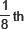of the radius of the original ball. Is it true?
(2 Marks)
3.   A metallic sphere of radius 4.2 cm is melted and recast into the shape of a cylinder of radius 6 cm.
Find the height of the cylinder.
(3 Marks)
4.   The figure shows a decorative block which is made of two solids a cube and a hemisphere. The base of the block is a cube with edge 5 cm and the hemisphere, fixed on the top, has a diameter of 4.2 cm. Find the total surface area of the block.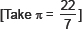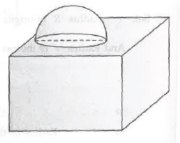(3 Marks)
5.   A bucket is in the form of a frustum of a cone. its depth is 15 cm and the diameters of the top and the bottom are 56 cm and 42 cm respectively. Find how much water can the bucket hold?
(3 Marks)
6.   The height of a cone is 30 cm. A small cone is cut off at the top by a plane parallel to the base. If its volume be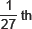of the volume of the given cone, at what height abvoe the base is the section made?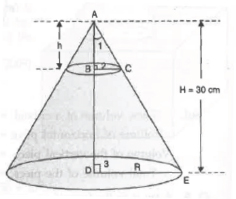(4 Marks)
7.   A hollow cone is cut by a plane parallel to the base and the upper portion is removed. If the curved surface of the remainder is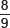of the curved surface of the whole cone, find the ratio of the line segments into which the altitude of the cone is divided by the plane.
(5 Marks)
8.   A circus tent is cylinderical to a height of 3 m and conical above it. If its base radius is 52.5 m and slant height of th econical portion is 53 m, find the area of the canvas needed to make the tent.
(4 Marks)